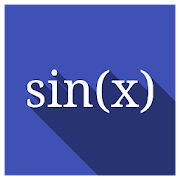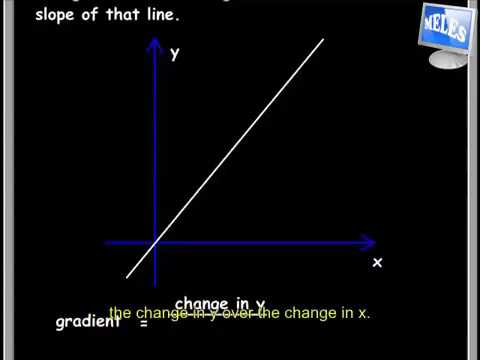# Trigonometry 1 FREE A-Level Pure MathEveryone
45ALL OUR 20 PURE MATH APPS ARE NOW 100% FREE!

★ Study your Pure Mathematics on the go; bus, café, beach, street, anywhere!

★ Simplified explanations, plus extra side notes with even more explanation!

★ Over 30 examples per chapter with step by step working.

★ Past paper examination questions at the end of each chapter.

★ Fully worked-out answers to every exercise per chapter are also available.

✪ Check out our publication series here:

✪ Featured Pure Mathematics chapters:

1. Differentiation 1
2. Differentiation 2
3. Integration
4. Further differentiation
5. Algebra 1
6. Binomial theorem
7. Algebra 2
8. Series
9. Trigonometry 1
10. Trigonometry 2

11. Further Integration 1
12. Exponential and Log functions
13. Partial fractions
14. Further Integration 2
15. Coordinate geometry 1
16. Curve sketching
17. Coordinate geometry 2
18. Differential equations
19. Complex numbers
20. Vectors in 3-dimensions

✪ We've created an app for every Pure Maths chapter above.

✪ This app is for Chapter 9 referred to as TRIGONOMETRY 1 and is 100% FREE for your study.
Collapse

Review Policy
4.7
45 total
5
4
3
2
1

## What's New

ALL OUR PREMIUM APPS ARE NOW 100% FREE!
Study on your tablet and smart TV:
✸ Trigonometric ratios of angles
✸ Graphs of sinθ, cosθ and tanθ
✸ Trigonometric ratios of 30º, 45º and 60º
✸ Secant, cosecant and cotangent
✸ Trigonometric equations
✸ Pythagoras theorem
✸ Compound angle formula
✸ Double angle formula
✸ The t–formula
✸ The form acosθ + bsinθ
✸ The factor formulae
✸ The sine and cosine rule
✸ The area of a triangle
Collapse

Updated
July 12, 2020
Size
6.8M
Installs
10,000+
Current Version
2.0
Requires Android
4.4 and up
Content Rating
Everyone
Permissions
Offered By
Multimedia E-Learning Education System (MELES)
Developer
KSDAH P.O. Box 391 Kanye, BWSee more